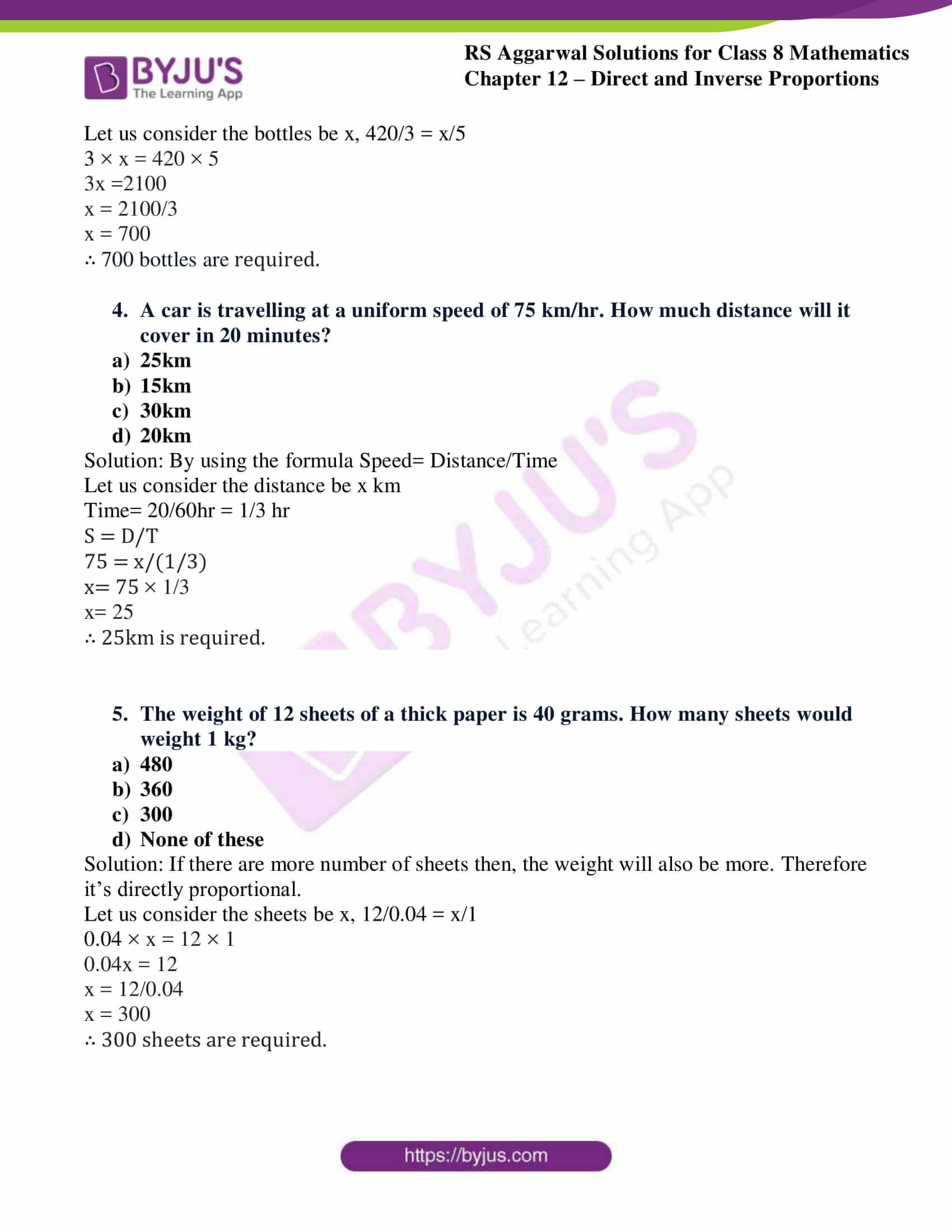# RS Aggarwal Solutions for Class 8 Maths Chapter 12 - Direct and Inverse Proportions Exercise 12C

RS Aggarwal Solutions for Class 8 Maths Chapter 12- Exercise 12C, Direct and Inverse Proportions links are provided below, students can refer and download them. BYJU’S expert team has solved the RS Aggarwal Solutions to ensure that the students are thorough with their basic concepts by practicing the solutions.

In Exercise 12C of RS Aggarwal Class 8 Maths, we shall brief all the topics covered in Chapter 12.

## Download PDF of RS Aggarwal Solutions for Class 8 Maths Chapter 12- Direct and Inverse Proportions Exercise 12C### Access Answers to RS Aggarwal Solutions for Class 8 Maths Chapter 12- Direct and Inverse Proportions Exercise 12C

Select the correct answer in each of the following:

1. If 14 kg of pulses cost ₹882, what is the cost of 22 kg of pulses?

1. ₹1254
2. ₹1298
3. ₹1342
4. ₹1386

Solution: If more amount of pulses, the cost will be more. Therefore it’s directly proportional.

Let us consider the cost be x, 882/14 = x/22

14 × x = 882 × 22

14x = 19404

x = 19404/14

x = 1386

∴ ₹1386 is the cost

2. If 8 orange cost ₹52, how many oranges can be bought for ₹169?

1. 13
2. 18
3. 26
4. 24

Solution: If more amount of oranges, the cost will be more. Therefore it’s directly proportional.

Let us consider the amount be x, 8/52 = x/169

52 × x = 8 × 169

52x = 1352

x = 1352/52

x = 26

∴ 26 oranges can be bought.

3. A machine fills 420 bottles in 3 hours. How many bottles will it fill in 5 hours?

1. 252
2. 700
3. 504
4. 300

Solution: If more number of
bottles required then, time taken will also be more. Therefore it’s directly proportional.

Let us consider the bottles be x, 420/3 = x/5

3 × x = 420 × 5

3x =2100

x = 2100/3

x = 700

∴ 700 bottles are required.

4. A car is travelling at a uniform speed of 75 km/hr. How much distance will it cover in 20 minutes?

1. 25km
2. 15km
3. 30km
4. 20km

Solution: By using the formula Speed= Distance/Time

Let us consider the distance be x km

Time= 20/60hr = 1/3 hr

S = D/T

75 = x/(1/3)

x= 75 × 1/3

x= 25

∴ 25km is required.

5. The weight of 12 sheets of a thick paper is 40 grams. How many sheets would weight 1 kg?

1. 480
2. 360
3. 300
4. None of these

Solution: If there are more number of sheets then, the weight will also be more. Therefore it’s directly proportional.

Let us consider the sheets be x, 12/0.04 = x/1

0.04 × x = 12 × 1

0.04x = 12

x = 12/0.04

x = 300

∴ 300 sheets are required.

### Access other Exercises of RS Aggarwal Solutions for Class 8 Maths Chapter 12- Direct and Inverse Proportions

Exercise 12A Solutions 15 Questions

Exercise 12B Solutions 15 Questions

## RS Aggarwal Solutions for Class 8 Maths Chapter 12 – Direct and Inverse Proportions Exercise 12C

Exercise 12C of RS Aggarwal Class 8, Direct and Inverse Proportions. This exercise mainly deals with the Objective Questions related to the topics discussed earlier in Chapter 12.

Students are suggested to try solving the questions from RS Aggarwal book of Class 8 and then refer to these solutions to identify the best way of approaching the different questions. By referring to these solutions, students develop problem-solving abilities and can tackle any type of questions easily. Thus, practicing as many times as possible helps in building time management skills and also boosts the confidence level to achieve high marks.